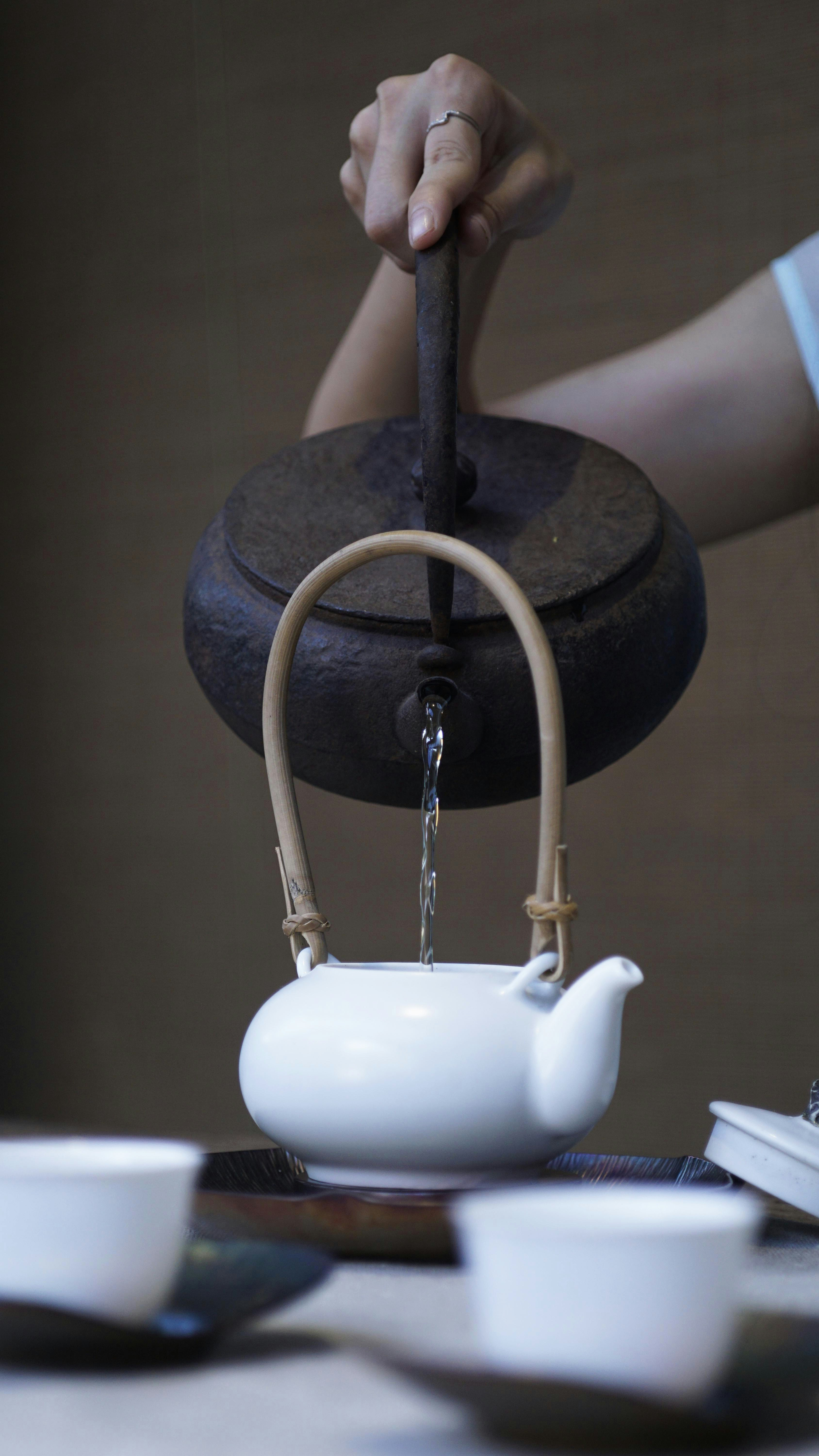Hackerrank Mathematics- Die Hard 3 Solution

# Hackerrank Mathematics- Die Hard 3 SolutionSimon: On the fountain, there should be 2 jugs - a 5 gallon and a 3 gallon. Do you see them? Fill one of the jugs with exactly 4 gallons of water and place it on the scale and the timer will stop. You must be precise; one ounce more or less will result in detonation. If you’re still alive in 5 minutes, we’ll speak.

Bruce: Wait, wait a second. I don’t get it. Do you get it?

Samuel: No.

Bruce: Get the jugs. Obviously, we can’t ﬁll the 3 gallon jug with 4 gallons of water.

Samuel: Obviously.

Bruce: All right. I know, here we go. We ﬁll the 3 gallon jug exactly to the top, right?

Samuel: Uh huh.

Bruce: Okay, now we pour this 3 gallons into the 5 gallon jug, giving us exactly 3 gallons in the 5 gallon jug, right?

Samuel: Right, then what?

Bruce: We take the 3 gallon jug and ﬁll it a third of the way...

Samuel: No! He said, “Be precise.” Exactly 4 gallons.

Bruce: Damn! Every cop within 50 miles is running his ass off and I’m out here playing a kids' games in the park.

Samuel: Hey, you want to focus on the problem at hand?

Given 2 jugs of capacity a and b gallons, and an infinite supply of water, can you fill one of the jugs with exactly c gallons of water ?

Input Format

First line contains the number of testcases T. T lines follow.
Each line contains 3 space separated integers a, b and c . a and b indicate the capacity of the two jugs respectively, and c denotes the exact capacity with which one of the jugs should be filled.

Output Format

For each test case, print "YES" (in a new line) if one of the jugs can be filled with exactly c gallons of water and "NO" (in a new line) if they cannot be filled. ( quotes are for clarity )

Constraints

1 ≤ a, b, c ≤ 103
1 ≤ T ≤ 100

Sample Input

2
5 3 4
3 6 4


Sample Output

YES
NO


Explanation

Bruce can do the following, fill jug a with 5 gallons.

a = 5, b = 0


Now, he can fill jug b with 3 gallons from jug a.

a = 2, b = 3


He can empty jug b and empty 2 gallons from jug a to jug b.

a = 0, b = 2


Now, he can fill jug a with 5 gallons and fill jug b with 1 gallon from jug a. This results in jug a containing exactly 4 gallons of water.

a = 5, b = 2
a = 4, b = 3


In the second testcase, it is impossible to generate 4 gallons of water, hence NO.

### Solution in Python

def solve(a,b,c):
A= a
x= 0
while True:
x = a-(b-x)
if x==A:
return "NO"
if x==c:
return "YES"
while(x>b):
x = x-b
if x==c:
return "YES"

for i in range(int(input())):
a, b , c= map(int, input("").split())
x = [a,b]
x.sort()
b,a = x
print(solve(a,b,c))